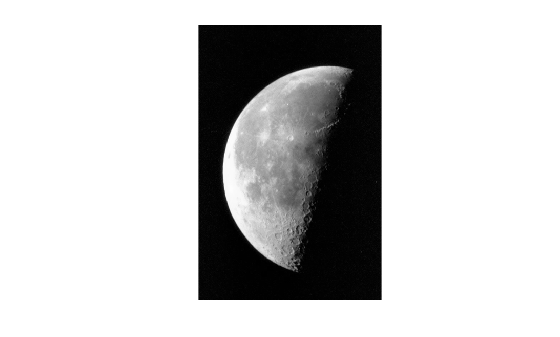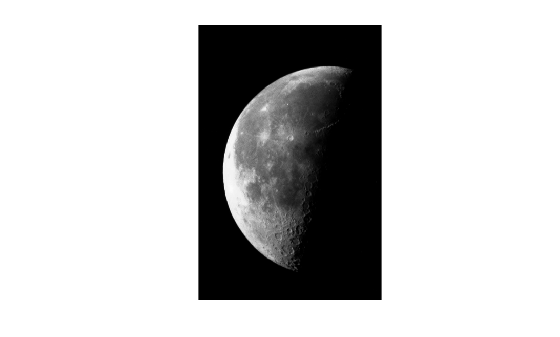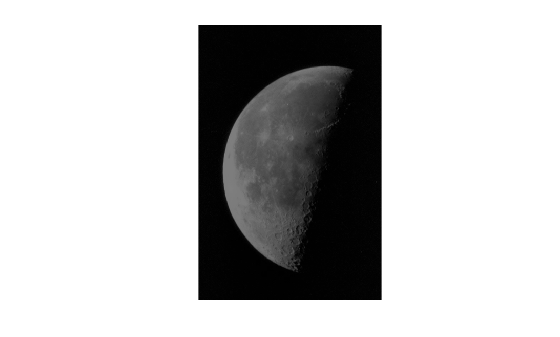# immultiply

Multiply two images or multiply image by constant

## Syntax

``Z = immultiply(X,Y)``

## Description

example

````Z = immultiply(X,Y)` multiplies each element in array `X` by the corresponding element in array `Y` and returns the product in the corresponding element of the output array `Z`. ```

## Examples

collapse all

Read a grayscale image into the workspace, then convert the image to `uint8`.

```I = imread('moon.tif'); I16 = uint16(I);```

Multiply the image by itself. Note that `immultiply` converts the class of the image from `uint8` to `uint16` before performing the multiplication to avoid truncating the results.

`J = immultiply(I16,I16);`

Show the original image and the processed image.

`imshow(I)````figure imshow(J)```Read an image into the workspace.

`I = imread('moon.tif');`

Scale each value of the image by a constant factor of 0.5.

`J = immultiply(I,0.5);`

Display the original image and the processed image.

`imshow(I)````figure imshow(J)```## Input Arguments

collapse all

First array, specified as a numeric array or logical array of any dimension.

Second array to be multiplied with `X`, specified as a numeric scalar, numeric array, or logical array.

• If `X` is numeric, then the size and class of `Y` can have one of the following values:

• `Y` is the same size and class as `X`.

• `Y` is the same size as `X` and is logical.

• `Y` is a scalar of type `double`.

• If `X` is logical, then `Y` must have the same size as `X`. `Y` can be any class.

## Output Arguments

collapse all

Product, returned as a numeric array.

• If `X` is numeric, then `Z` has the same size and class as `X`.

• If `X` is logical, then `Z` has the same size and class as `Y`.

`immultiply` computes each element of `Z` individually in double-precision floating point. If `X` or `Y` is an integer array, then elements of `Z` exceeding the range of the integer type are truncated, and fractional values are rounded.

## Tips

• If `X` and `Y` are numeric arrays of the same size and class, then you can use the expression `X.*Y` instead of `immultiply`.

## Version History

Introduced before R2006a